Request a call back

# NCERT Solutions for Class 10 Maths CBSE

Practise NCERT solutions for CBSE Class 10 Mathematics developed by qualified academic experts and prepare thoroughly for your board exams. TopperLearning’s solutions for National Council of Educational Research and Training (NCERT) books are used by students in search of reliable answers when they practice Maths. These solutions are created as per the latest CBSE Class 10 Maths syllabus.

Use the CBSE Class 10 NCERT solutions to compare your attempted answers with learn how to improve in the process. Your Class 10 syllabus consists of variety of topics – some may need you to apply logic, some may require creative thinking. And then, there will be questions where you will have to use your geometry tools. With the correct solutions at your disposal, you can fine-tune your skills.

The TopperLearning study portal is among the best places online to look for study material. Our chapter-wise NCERT CBSE Class 10 Maths solutions are accessed 24x7 by students like you for revising Maths concepts such as Euclid’s lemma, trigonometry, circles, surface area of a cylinder, and so on. The solutions for each chapter are published as per the exercise numbers in the textbooks and can be quickly accessed for last-minute revision.

You can go to the NCERT CBSE Class 10 Maths Textbook solutions of a specific chapter by selecting the chapter from our easy-to-use drop-down chapter list. Whether you want to revise an exercise in the chapter on arithmetic progressions or check out probability problems, we’ve got you covered. TopperLearning’s NCERT solutions allow you to improve your problem-solving techniques, revise formulae, and enable you to score more marks.

## NCERT Solutions for Class 10 Maths

NCERT solutions for Class 10 Maths Chapter 1 Real Numbers

The first chapter in CBSE Class 10 talks about HCF and LCM. This is followed by Euclid’s division lemma, rational and irrational numbers, and conversion of fractions to decimals and decimals to fractions. TopperLearning’s Class 10 Maths solutions have the solutions to all the questions present in all four exercises. These solutions help you to revise and practice with the easiest methods provided by our experts.

Fundamental Theorem of Arithmetic:

Every composite number can be expressed (factorized) as a product of primes, and this factorization is unique, apart from the order in which the prime factors occur.

The procedure of finding HCF(Highest Common Factor) and LCM(Lowest Common Multiple) of given two positive integers a and b:

Find the prime factorization of given numbers.

HCF (a, b) = Product of the smallest power of each common prime factors in the numbers.

LCM (a, b) = Product of the greatest power of each prime factors, involved in the numbers.

Relationship between HCF and LCM of two numbers:

If a and b are two positive integers, then HCF (a, b) × LCM (a, b) = a × b

Relationship between HCF and LCM of three numbers:Watch a word problem video on LCM and HCF, below.

Chapter 1- Real Numbers- Ex 1.1

Practise how to apply Euclid’s algorithm with our step-wise NCERT CBSE Class 10 Maths Chapter 1 Exercise 1.1 solutions. Get concept insights by experts on how to correctly apply Euclid’s division lemma in a tricky Maths problem. Also, check expert tips on how to solve word problems quickly.

Chapter 1- Real Numbers- Ex 1.2

Learn to work with exponents in a Maths problem on real numbers. Understand numbers better by recognising prime numbers. In our NCERT solutions, read the concept insights to understand when to express a number as the product of prime factors.

Chapter 1- Real Numbers- Ex 1.3

Revise problems based on rational numbers and irrational numbers. Understand what are co-prime integers. Find out how to solve a problem with proof by contradiction. With the concept insights given by TopperLearning’s academic experts, you will have better clarity of the CBSE Class 10 Maths NCERT solutions on real numbers.

Chapter1- Real Numbers- Ex 1.4

You may have heard of concepts like terminating and non-terminating decimal expansions. Learn how to identify these expansions and solve problems with our CBSE Class 10 Maths NCERT solutions. Also, understand the concept of prime factorisation.

NCERT Real Numbers Class 10 Videos

NCERT Real Numbers Class 10 Revision Notes

NCERT Real Numbers Class 10 Most Important Questions

NCERT Real Numbers Class 10 MCQ

NCERT Real Numbers Class 10 FIB

NCERT Real Numbers Class 10 Short Answer Questions

List of sub chapters

Class 10 Maths- Euclids division lemma and algorithm

Class 10 Maths- Fundamental theorem of arithmetic

Class 10 Maths- Rational and irrational numbers

NCERT solutions for Class 10 Maths Chapter 2 Polynomials

Chapter 2 of Class 10 CBSE is on polynomials. Our solutions tell you how to verify the relation between zeros and the coefficient. The NCERT solutions also explain the method used to find the zeros of polynomials and the solutions for quadratic polynomials.

What is a polynomial?

polynomial p(x) in one variable x is an algebraic expression in x of the form

p(x) =  anXn + an-1Xn-1 + an-2Xn-2 + ........ + a2X2 + a1X + a0, where x is a variable where x is a variable

a0, a1, a2 ....... an are respectively the coefficients of x0, x1, x2, x3, …… xn.

Each of  anXn , an-1Xn-1 , an-2Xn-2 , ......a2X2, a1X, a0 with an ≠ 0 is called the term of a polynomial.

The highest exponent of the variable in a polynomial determines the degree of the polynomial.

Types of polynomials

A polynomial of degree zero is called a constant polynomial. Examples: –9x0,.

A polynomial of degree one is called a linear polynomial. It is of the form ax + b.

Examples: x – 2, 4y + 89, 3x – z.

A polynomial of degree two is called a quadratic polynomial. It is of the form ax2 + bx + c, where a, b, c are real numbers and a ≠ 0.

Examples: x2 - 2x + 5, x2 – 3x etc.

A polynomial of degree 3 is called a cubic polynomial and has the general form ax3 + bx2 + cx +d. For example: x3 + 2x2 - 2x + 5 etc.

Value of the polynomial

If p(x) is a polynomial in x, and k is a real number then the value obtained after replacing x by k in p(x) is called the value of p(x) at x = k which is denoted by p(k).

Zero of a polynomial

A real number k is said to be the zero of the polynomial p(x), if p(k) = 0.

Zeroes of the polynomial can be obtained by solving the equation p(x) = 0.

It is possible that a polynomial may not have a real zero at all.

For any linear polynomial ax + b, the zero is given by the expression (–b/a) = – (constant term)/(Coefficient of x).

Number of zeroes of a polynomial

The number of real zeros of the polynomial is the number of times its graph touches or intersects x-axis.

The graph of a polynomial p(x) of degree n intersects or touches the x-axis at most n points.

A polynomial of degree n has at most n distinct real zeroes.

Watch a problem solving video below.

Chapter 2- Polynomials- Ex 2.1

So, how to find the number of zeroes in a polynomial? Practise the exercise problems and solutions to grasp the techniques for finding zeroes in a polynomial.

Chapter 2- Polynomials - Ex 2.2

Learn to calculate the product of zeroes and sum of zeroes by revising the problems and solutions in this section. Understand quadratic polynomials better by revising our expert solutions for CBSE Class 10 Maths NCERT exercises.

Chapter 2- Polynomials - Ex 2.3

Revise the division algorithm and learn to use it to calculate answers in exercises on polynomials. Practise the problems and CBSE Class 10 Maths solutions in this section to understand concepts such as dividend, remainder, and quotient.

Chapter 2- Polynomials - Ex 2.4

Dive deeper into the concept of zero of a polynomial and revisit the division algorithm by practising related CBSE Class 10 Maths problems. Understand how to work with factors of a polynomial by reviewing the steps by experts.

NCERT Polynomials Class 10 Videos

NCERT Polynomials Class 10 Revision Notes

NCERT Polynomials Class 10 Most Important Questions

NCERT Polynomials Class 10 MCQ

NCERT Polynomials Class 10 Short Answer Questions

List of sub chapters

Class 10 Maths- Geometric meaning of the zeroes

Class 10 Maths- Zeroes and coefficients

Class 10 Maths- Division algorithm for polynomials

NCERT solutions for Class 10 Maths Chapter 3 Pairs of Linear Equations in Two Variables

Class 10 Maths Chapter 3 is the study of algebraic and graphical methods for solving linear equations. With our solutions, revise the elimination and cross-multiplication method that can be used in solving problems based on linear equations.

1. The general form of a pair of linear equations in two variables is

a1x + b1y + c1 = 0

a2x + b2y + c2 = 0

where a1, a2, b1, b2, c1 and c2 are real numbers, such that

2. A system of linear equations in two variables represents two lines in a plane. For two given lines in a plane there could be three possible cases:

The two lines are intersecting, i. e., they intersect at one point.

The two lines are parallel, i.e., they do not intersect at any real point.

The two lines are coincident lines, i.e., one line overlaps the other line.

3. A system of simultaneous linear equations is said to be

Consistent, if it has at least one solution.

In-consistent, if it has no solution.

4. While solving problems based on time, distance and speed; following knowledge may be useful:

If speed of a boat in still water = u km/hr,

Speed of the current = v km/hr

Then,

Speed upstream = (u - v) km/hr

Speed downstream = (u + v) km/hr

Watch a problem solving video on this chapter.

Chapter 3 - Pairs of Linear Equations in Two Variables- Ex 3.1

Learn the steps to solve algebraic equations with our chapter solutions. TopperLearning’s NCERT textbook solutions will give you clarity on how to draw the correct graphs to represent the equations.

Chapter 3 - Pairs of Linear Equations in Two Variables- Ex 3.2

Revise the steps to represent values from equations in a table format with our Class 10 Maths solutions. Check the expert answers to identify the values of ‘x’ and ‘y’ co-ordinates of a point in a graph.

Chapter 3 - Pairs of Linear Equations in Two Variables- Ex 3.3

How to substitute a given value in an equation to provide proof or find an answer? Learn this and more by going through the step-wise CBSE Class 10 Maths Chapter 3 Exercise 3.3 solutions for questions from NCERT textbook.

Chapter 3 – Pairs of Linear Equations in Two Variables- Ex 3.4

Solve the exercises in your NCERT Maths textbook with help of the accurate solutions by qualified Maths teachers. Revise problem-solving methods such as elimination method and substitution method using the NCERT solutions.

Chapter 3 – Pairs of Linear Equations in Two Variables- Ex 3.5

Sometimes, the given pair of equations may have a unique solution. Knowing the cross-multiplication method will help you solve such questions. Practice related exercise questions with our CBSE Class 10 Maths NCERT textbook solutions.

Chapter 3 - Pairs of Linear Equations in Two Variables- Ex 3.6

How will you solve problems wherein two equations are not linear? Read our concept insights and practise CBSE Class 10 Maths Chapter 3 Exercise 3.6 solutions from this section to get the answers.

Chapter 3 - Pairs of Linear Equations in Two Variables- Ex 3.7

Learn to use algebraic methods to solve linear equations by practising the Maths problems and solutions from this section. Refer to the NCERT solutions, understand the application of chapter concepts to solve real problems using linear equations.

NCERT Pair of Linear Equations in two Variables Class 10 Videos

NCERT Pair of Linear Equations in two Variables Class 10 Revision Notes

NCERT Pair of Linear Equations in two Variables Class 10 Most Important Questions

NCERT Pair of Linear Equations in two Variables Class 10 MCQ

NCERT Pair of Linear Equations in two Variables Class 10 FIB

NCERT Pair of Linear Equations in two Variables Class 10 Short Answer Questions

List of sub chapters

Class 10 Maths- Graphical solution to linear equations

Class 10 Maths- Substitution method

Class 10 Maths- Elimination method

Class 10 Maths- Cross multiplication method

Class 10 Maths- Equations reducible to linear form

NCERT solutions for Class 10 Maths Chapter 4 Quadratic Equations

Chapter 4 is about converting word problems to quadratic equations. By using our expert answers or solutions to your Maths-related questions as your reference, you will be able to find the roots of a quadratic equation in this chapter.

1. Introduction to Quadratic equation

If p(x) is a quadratic polynomial, then p(x) = 0 is called a quadratic equation.

The general or standard form of a quadratic equation, in the variable x, is given by ax2 + bx + c = 0, where a, b, c are real numbers and a ≠ 0.

2. Roots of the quadratic equation

The value of x that satisfies an equation is called the zeroes or roots of the equation.

A real number α is said to be a solution/root of the quadratic equation ax2 + bx + c = 0 if aα2 + bα + c = 0.

A quadratic equation has at most two roots.

A quadratic equation can be solved by following algebraic methods:

Splitting the middle term (factorization)

Completing squares

The roots of a quadratic equation ax2 + bx + c = 0 can be calculated by using the quadratic formula:If b2 – 4ac < 0, then equation does not have real roots.

Please watch a problem solving video here.

Chapter 4 - Quadratic Equations- Ex 4.1

How to prove that a given equation is a quadratic equation? You can find answers to quadratic equations concepts part of your syllabus and proofs in our CBSE Class 10 Maths Chapter 4 solutions.

Chapter 4 - Quadratic Equations- Ex 4.2

While solving quadratic equations, you may have to use various techniques such as split the middle term method, Pythagoras theorem, etc. Learn to apply these techniques by learning from TopperLearning’s Class 10 Maths solutions.

Chapter 4 - Quadratic Equations- Ex 4.3

In quadratic equations, learn to apply logic such as the square of a number cannot be negative and other concepts. Our CBSE Class 10 Maths solutions equip you with concept insights to understand and apply logic while writing Maths answers.

Chapter 4 - Quadratic Equations- Ex 4.4

Solve the NCERT exercise for Chapter 4 to understand how to work with discriminants for find the roots in a given equation. With the solutions, grasp the ways to find answers to real problems such as finding the perimeter of a fence or length of a park.

NCERT Quadratic Equations Class 10 Videos

NCERT Quadratic Equations Class 10 Revision Notes

NCERT Quadratic Equations Class 10 Most Important Questions

NCERT Quadratic Equations Class 10 MCQ

NCERT Quadratic Equations Class 10 FIB

NCERT Quadratic Equations Class 10 Short Answer Questions

List of sub chapters

Class 10 Maths- Solution of quadratic equations by factorisation

Class 10 Maths- Solving quadratic equations using the formula

Class 10 Maths- Method of completing the square

Class 10 Maths- Nature of roots

Class 10 Maths- Word problems in quadratic equations

NCERT solutions for Class 10 Maths Chapter 5 Arithmetic Progressions

Chapter 5 has questions on arithmetic progressions. To help you understand the concept of arithmetic progression, our experts have shared detailed answers to the NCERT textbook questions in our NCERT Solutions for Class 10.

Arithmetic Progression:

An arithmetic progression is a list of numbers in which each term is obtained by adding a fixed number to the preceding term, except the first term.

Each of the numbers of the sequence is called a term of an Arithmetic Progression. The fixed number is called the common difference. This common difference could be a positive number, a negative number or even zero.

General form and general term (nth  term) of an A.P:

The general form of an A.P. is a, a + d, a + 2d, a + 3d….., where ‘a’ is the first term and ‘d’ is the common difference.

The general term (nth term) of an A.P is given by an = a + (n - 1)d, where ‘a’ is the first term and ‘d’ is the common difference.

Sum of n terms of an A.P:

Sum of n terms of an A.P. is given bywhere ‘a’ is the first term, ‘d’ is the common difference and ‘n’ is the total number of terms.

Sum of n terms of an A.P. is also given by:where ‘a’ is the first term and ‘’ is the last term.

Sum of first n natural numbers is given by.

The nth term of an A.P is the difference of the sum to first n terms and the sum to first (n -1) terms of it. That is, an = Sn - Sn-1

Watch a problem solving video on AP, below.

Chapter 5 - Arithmetic Progressions- Ex 5.1

Along with the CBSE Class 10 Maths NCERT solutions, get the best tips to present the arithmetic progression as per given data. Brush up your basics by going through the solved exercises in this section.

Chapter 5 - Arithmetic Progressions- Ex 5.2

Revisit concepts such as First Term, Common Difference, etc. by browsing through the solutions for Chapter 5 exercise questions. Learn to find the missing terms in a given sequence by studying from our CBSE Class 10 Maths Chapter 5 Exercise 5.2 solutions.

Chapter 5 - Arithmetic Progressions- Ex 5.3

Understand how to work out the sum of first n terms with the appropriate formula. Learn to find the required values in a problem by multiplying or subtracting equations. Revise the NCERT model answers for Class 10 to grasp ways to analyse the given data for finding the required answers.

Chapter 5 - Arithmetic Progressions- Ex 5.4

How to find the first negative term in an arithmetic progression? How to use the sum of n terms formula to find the value of certain objects that are placed uniformly in an AP format? To find answers to these queries, solve the Chapter 5 exercise questions and review the model answers.

NCERT Arithmetic Progression Class 10 Videos

NCERT Arithmetic Progression Class 10 Revision Notes

NCERT Arithmetic Progression Class 10 Most Important Questions

NCERT Arithmetic Progression Class 10 MCQ

NCERT Arithmetic Progression Class 10 FIB

NCERT Arithmetic Progression Class 10 Short Answer Questions

List of sub chapters

Class 10 Maths Introduction to Arithmetic Progression

Class 10 Maths nth term of an Arithmetic Progression

Class 10 Maths Sum of n terms of an Arithmetic Progression

Class 10 Maths Word problems of Arithmetic Progression

NCERT solutions for Class 10 Maths Chapter 6 Triangles

Chapter 6 includes solutions on the important topic of triangles. Understand how the shape of a triangle is formed. Solve problems related to the properties of triangles in this chapter. Study the different types of triangles such as isosceles triangle, right-angled triangle, and equilateral triangle, and learn about applications of triangles in trigonometry with Class 10 Maths solutions.

Basic Proportionality Theorem (Thales Theorem):

If a line is drawn parallel to one side of a triangle to intersect other two sides in distinct points, the other two sides are divided in the same ratio.

Converse of BPT:

If a line divides any two sides of a triangle in the same ratio then the line is parallel to the third side.

A line drawn through the mid-point of one side of a triangle which is parallel to another side bisects the third side. In other words, the line joining the mid-points of any two sides of a triangle is parallel to the third side.

AAA (Angle-Angle-Angle) similarity criterion:

If in two triangles, corresponding angles are equal, then their corresponding sides are in the same ratio (or proportion) and hence the two triangles are similar.

AA (Angle-Angle) similarity criterion:

If two angles of a triangle are respectively equal to two angles of another triangle, then by the angle sum property of a triangle their third angles will also be equal.

Thus, AAA similarity criterion changes to AA similarity criterion which can be stated as follows:

If two angles of one triangle are respectively equal to two angles of other triangle, then the two triangles are similar.

Converse of AAA similarity criterion:

If two triangles are similar, then their corresponding angles are equal.

SSS (Side-Side-Side) similarity criterion:

If in two triangles, sides of one triangle are proportional to (i.e., in the same ratio of) the sides of the other triangle, then their corresponding angles are equal and hence the two triangles are similar.

Converse of SSS similarity criterion:

If two triangles are similar, then their corresponding sides are in constant proportion.

SAS (Side-Angle-Side) similarity criterion:

If one angle of a triangle is equal to one angle of the other triangle and the sides including these angles are proportional, then the two triangles are similar.

Converse of SAS similarity criterion:

If two triangles are similar, then one of the angles of one triangle is equal to the corresponding angle of the other triangle and the sides including these angles are in constant proportion.

Pythagoras Theorem:

In a right triangle, the square of the hypotenuse is equal to the sum of the squares of the other two sides.

Thus, in triangle ABC right angled at B, AB2 + BC2 = AC2

Converse of Pythagoras Theorem:

If in a triangle, square of one side is equal to the sum of the squares of the other two sides, then the angle opposite the first side is a right angle.

The ratio of the areas of two similar triangles is equal to the square of the ratio of their corresponding sides.

Thus, if Δ ABC ~ Δ PQR, then.

Also, the ration of the areas of two similar triangles is equal to the ration of the squares of the corresponding medians.

Chapter 6 - Triangles- Ex 6.1

Learn how to construct equilateral triangles, parallelograms, squares and trapeziums. Brush up your knowledge of triangles with our NCERT solutions.

Chapter 6 - Triangles- Ex 6.2

Where and how would you use the basic proportionality theorem? Get the answers in the CBSE Class 10 Maths Exercise 6.2 solutions to exercise questions in this section.

Chapter 6 - Triangles- Ex 6.3

Learn about similarity of triangles by reviewing the expert solutions to some of the Chapter 6 exercises. Understand the Angle-Angle-Angle (AAA) rule of triangles with the help of TopperLearning’s NCERT solutions.

Chapter 6 - Triangles- Ex 6.4

Discover the concepts such as vertically opposite angles and alternate interior angles to better understand the properties of a triangle. Revise the various rules of triangles such as Side-Side-Side (SSS) and more with our NCERT textbook solutions.

Chapter 6 - Triangles- Ex 6.5

Through these CBSE Class 10 Maths Chapter 6 NCERT solutions, revise the properties of a right-angled triangle. Also, practise the usage of Pythagoras theorem to write the perfect answers.

Chapter 6 - Triangles- Ex 6.6

Use the NCERT solutions for Chapter 6 to revise problems based on isosceles triangles. Revisit different types of problems wherein you understand the properties of triangles, which are part of a quadrilateral or a circle.

NCERT Arithmetic Progression Class 10 Videos

NCERT Triangles Class 10 Videos

NCERT Triangles Class 10 Revision Notes

NCERT Triangles Class 10 Most Important Questions

NCERT Triangles Class 10 MCQ

NCERT Triangles Class 10 FIB

NCERT Triangles Class 10 Short Answer Questions

List of sub chapters

Class 10 Maths- Basic proportionality theorem

Class 10 Maths- Converse of BPT

Class 10 Maths- Criteria for similar triangles

Class 10 Maths- Areas of similar triangles

Class 10 Maths-  Pythagoras theorem

Class 10 Maths- Problems on Pythagoras theorem

NCERT solutions for Class 10 Maths Chapter 7 Coordinate Geometry

Class 10 Maths Chapter 7 improves your knowledge about concepts such as slopes, origins, axes, coordinates etc. In this chapter, you will learn how to find the coordinates as per the given data. Explore answers such as finding the distance between two points, using their coordinates, and more.

1. If P is a point lying on AB produced such that AP: BP = m: n, then point P divides AB externally in the ratio m:n.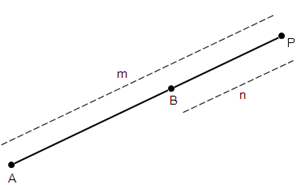If P divides the line segment joining the points A(x1, y1)and B(x2, y2) in the ratio m: n externally, then the coordinates of point P are given by.

2. Coordinates of Mid-point

Mid-point divides the line segment in the ratio 1:1. Coordinates of the mid-point of a line segment joining the points (x1, y1) and (x2, y2) areWatch a quick problem solving video on this chapter, below.

Chapter 7 - Coordinate Geometry - Ex 7.1

Practise problems on finding the distance between two points with our CBSE Class 10 Maths Chapter 5 Exercise 7.1 solutions. Revise the properties of quadrilaterals by revising chapter-specific problems.

Chapter 7 - Coordinate Geometry - Ex 7.2

Revise the accurate steps to use the section formula and find the coordinates of a given point. Use TopperLearning’s NCERT solutions to understand how to find the points part of the trisection of a given line segment.

Chapter 7 - Coordinate Geometry - Ex 7.3

Learn when to consider the absolute value in a given problem by reading the concept insights in our NCERT solutions. Understand the relation between collinear points and a triangle. Also, revise the steps to use area of a triangle to calculate the area of a quadrilateral.

Chapter 7 - Coordinate Geometry - Ex 7.4

Revise the CBSE Class 10 Maths NCERT exercises and solutions in Chapter 7 to understand calculations that help in finding the centre of a circle. Learn to prove that a given figure is a rhombus. Also, revise how to use the median of a triangle to calculate coordinates in a given problem.

NCERT Coordinate Geometry Class 10 Videos

NCERT Coordinate Geometry Class 10 Revision Notes

NCERT Coordinate Geometry Class 10 Most Important Questions

NCERT Coordinate Geometry Class 10 MCQ

NCERT Coordinate Geometry Class 10 Short Answer Questions

List of sub chapters

Class 10 Maths- Distance formula

Class 10 Maths- Section formula

Class 10 Maths- Area of triangles

NCERT solutions for Class 10 Maths Chapter 8 Introduction to Trigonometry

Take advantage of our well-written NCERT solutions for Class 10 Maths Chapter 8 to practice problems related to trigonometry. Understand how to tackle problems on trigonometric ratios of angles from NCERT Solutions.

Trigonometric Ratios

Ratio of the sides of a right triangle with respect to the acute angles is called the trigonometric ratios of the angle.

Trigonometric ratios of the acute angle A in right triangle ABC are given as follows: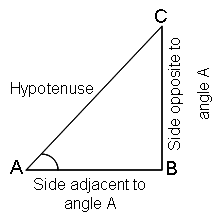Relation between trigonometric ratios:

The ratios cosec A, sec A and cot A are the reciprocals of the ratios sin A, cos A and tan A respectively as given:Trigonometric ratios of complementary angles:

Two angles are said to complementary angles if their sum is equal to 90°. Based on this relation, the trigonometric ratios of complementary angles are given as follows:

sin (90° – A) = cos A

cos (90° – A) = sin A

tan (90° – A) = cot A

cot (90° – A) = tan A

sec (90° – A) = cosec A

cosec (90° – A) = sec A

Note: tan 0° = 0 = cot 90°, sec 0° = 1 = cosec 90°. sec 90°, cosec 0°, tan 90° and cot 0° are not defined.

Basic trigonometric identities

sin2 θ + cos2 θ = 1

1 + tan2 θ = sec2 θ; 0 ≤ θ < 90°

1 + cot2 θ = cosec2 θ; 0 ≤ θ < 90°

Watch this video on trigonometric identities.

Chapter 8 - Introduction to Trigonometry - Ex 8.1

Revise how to use the Pythagoras theorem and trigonometric ratios to calculate the answers. Utilise the NCERT solutions and learn to read the given data accurately to calculate values of sin, cot, cos, tan and so on.

Chapter 8 - Introduction to Trigonometry - Ex 8.2

Go through the Chapter 8 exercise questions and the NCERT solutions created by experienced teachers to help you prepare for your exam. Understand the various methods to quickly solve complex problems on trigonometric ratios.

Chapter 8 - Introduction to Trigonometry - Ex 8.3

With the help of our chapter solutions for NCERT textbook exercises, learn to use existing values of a triangle and provide proofs as required. Also, understand the steps to calculate the value of the angle of a triangle using the relevant trigonometric formula.

Chapter 8 - Introduction to Trigonometry - Ex 8.4

In this section, practise exercises from the NCERT textbook wherein you have to prove that the given equation in the LHS is equal to RHS. Practise the CBSE Class 10 Maths Exercise 8.4 solutions multiple times to grasp the thought process for providing such proofs.

NCERT Introduction to Trigonometry Class 10 Videos

NCERT Introduction to Trigonometry Class 10 Revision Notes

NCERT Introduction to Trigonometry Class 10 Most Important Questions

NCERT Introduction to Trigonometry Class 10 MCQ

NCERT Introduction to Trigonometry Class 10 Short Answer Questions

List of sub chapters

Class 10 Maths- Trigonometrical ratios

Class 10 Maths- Trigonometric tables

Class 10 Maths- Trigonometric ratios of complementary angles

Class 10 Maths- Introduction to trigonometric identities

Class 10 Maths-  Applications of trigonometric identities

NCERT solutions for Class 10 Maths Chapter 9 Some Applications of Trigonometry

Get detailed answers or solutions on applications of trigonometry as per the latest CBSE Class 10 NCERT books. Learn to solve questions on finding the height and distance of various objects. Also, revise the various applications of trigonometry with our NCERT solutions for Class 10 Maths Chapter 9.

Angles of elevation and depression

The angle of elevation of an object viewed is the angle formed by the line of sight with the horizontal when it is above the horizontal level, i.e., the case when we raise our head to look at the object.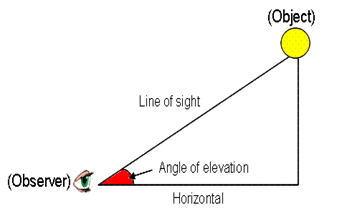The angle of depression of an object viewed is the angle formed by the line of sight with the horizontal when it is below the horizontal level, i.e., the case when we lower our head to look at the object.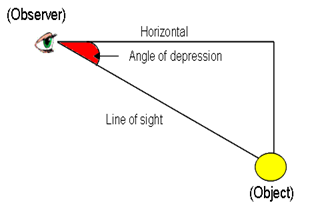Pythagoras theorem

It states that “In a right triangle, square of the hypotenuse is equal to the sum of the square of the other two sides”.

When any two sides of a right triangle are given, its third side can be obtained by using Pythagoras theorem.

Reflection from the water surface

In case of reflection from the water surface, the two heights above and below the ground level are equal in length.

Watch this video to understand an application problem on application of trigonometry

Chapter 9 - Some Applications of Trigonometry - Ex 9.1

Make use of our CBSE Class 10 Maths NCERT solutions to apply the correct steps for using trigonometry to solve real-life problems. In the exercise questions, you will get to learn how to calculate a distance travelled by a person or the height of a pole by applying the chapter concepts.

NCERT Some Applications of Trigonometry Class 10 Videos

NCERT Some Applications of Trigonometry Class 10 Revision Notes

NCERT Some Applications of Trigonometry Class 10 Most Important Questions

NCERT Some Applications of Trigonometry Class 10 MCQ

NCERT Some Applications of Trigonometry Class 10 Short Answer Questions

List of sub chapters

Class 10 Maths Introduction to heights and distances

Class 10 Maths Applications of heights and distances

NCERT solutions for Class 10 Maths Chapter 10 Circles

Understand concepts such as tangent, secant, and number tangents from a point to a circle and more with a proper solution. Also, learn about the relevance of the radius, diameter, and the centre while studying the topic of Circles in detail.

Perpendicular drawn from the centre of circle to a chord bisects the chord.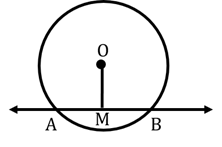A chord passing through the centre of the circle is called diameter of the circle.

A line segment joining the centre and a point on the circle is called radius of the circle.

A line which intersects a circle at two distinct points is called a secant of the circle.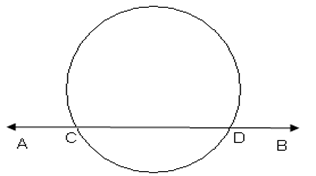A tangent to the circle is a line that intersects the circle (touches the circle) at only one point.

At any point on the circle, there can be one and only one tangent possible.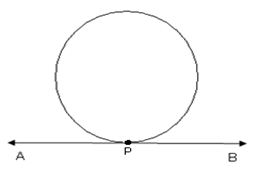The tangent at any point of a circle is perpendicular to the radius through the point of contact.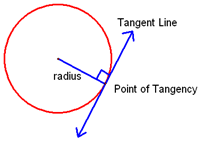The lengths of tangents drawn from an external point to the circle are equal.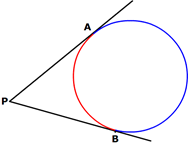Watch a problem solving video on this chapter

Chapter 10 - Circles - Ex 10.1

Revise the questions on the properties of circles through TopperLearning’s solutions for CBSE Class 10 Maths NCERT textbook exercises. Revisit the construction of tangents and secants. Also, learn to provide the necessary evidence to prove that the two given lines are parallel to each other.

Chapter 10 - Circles - Ex 10.2

Use our CBSE Class 10 Maths Exercise 10.2 solutions from the textbook to revise the important properties of triangles and circles. Learn to apply the Side-Side-Side Congruence rule in a given problem. Also, revise the properties of tangents and find out how you can write answers related to a quadrilateral that is circumscribing a circle.

NCERT Circles Class 10 Videos

NCERT Circles Class 10 Revision Notes

NCERT Circles Class 10 Most Important Questions

NCERT Circles Class 10 MCQ

NCERT Circles Class 10 Short Answer Questions

List of sub chapters

Class 10 Maths- Tangents to a circle

Class 10 Maths- Properties of tangents

NCERT solutions for Class 10 Maths Chapter 11 Constructions

Class 10 Maths Chapter solutions will help you to attempt questions that involve drawing a tangent to a circle. Also, find answers on accurately creating a division of the line segments in our textbook solutions. Additionally, you will learn how to give the mathematical justification or solution of a construction.

To divide a line segment internally in a given ratio m: n, where both m and n are positive integers, we follow the steps given below:

Step 1: Draw a line segment AB of given length by using a ruler.

Step 2: Draw any ray AX making an acute angle with AB.

Step 3: Along AX mark off (m + n) points A1, A2,………Am-1, Am+1,………,Am+n, such that AA1 = A1A2 = Am+n-1 Am+n.

Step 4: Join BAm+n

Step 5: Through the point Am, draw a line parallel to Am+nB by making an angle equal to AAm+nB at  Am, intersecting AB at point P.

The point P so obtained is the required point which divides AB internally in the ratio m:n.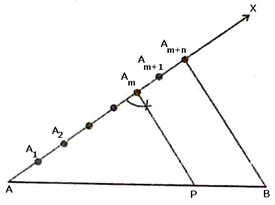Justification

In ΔABAm+n we observe that AmP is parallel to Am+nB. Therefore, by Basic Proportionality theorem, we have:Hence, P divides AB in the ratio m: n.

Watch a video on this chapter.

Chapter 11 - Constructions - Ex 11.1

Learn to construct line segments to complex figures as per given information. Benefit from the NCERT model answers by Maths experts. These CBSE Class 10 Maths NCERT solutions give you step-wise answers to textbook exercises on accurately constructing the required diagram.

Chapter 11 - Constructions - Ex 11.2

You can easily score marks in your board exam with an accurately constructed diagram. All you need is the guidance to create it step by step. In our class 10 maths solutions, you will get the required help to draw complex diagrams involving circles, triangles, tangents, etc.

NCERT Constructions Class 10 Videos

NCERT Constructions Class 10 Revision Notes

NCERT Constructions Class 10 Most Important Questions

NCERT Constructions Class 10 MCQ

NCERT Constructions Class 10 Short Answer Questions

List of sub chapters

Class 10 Maths- Division of a line segment

Class 10 Maths- Constructions of similar triangles

Class 10 Maths- Construction of tangents

NCERT solutions for Class 10 Maths Chapter 12 Areas Related to Circles

There are three exercises related to the area of circles in this chapter. Solve problems related to ‘Perimeter and Area of a Circle’, ‘Areas of Combinations of Plane Figures’ and ‘Areas of Sector and Segment of a Circle’. Use our NCERT solutions for Class 10 Maths Chapter 12 to revise this section thoroughly.

The region bounded by an arc of a circle and two radii at its end points is called a sector.

If the central angle of a sector is more than 180°, then the sector is called a major sector and if the central angle is less than 180°, then the sector is called a minor sector.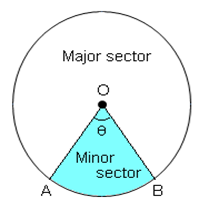Perimeter of sector of angle θ =Area of a sector of angle θ =Area of major sector = πr2 – Area of minor sector

Perimeter of segment of angle θ =Area of minor segment = Area of sector - Area of ΔABC

Area of major segment = Area of the circle – Area of minor segment

Watch an application video on this chapter, below.

Chapter 12 - Areas Related to Circles - Ex 12.1

Not sure how to use the circumference of a circle formula or the radius formula? Practise the answers to a variety of NCERT textbook exercises and learn how to use the right formula to find the area of a circle. Also, revise problems on finding the area of circular objects in real life.

Chapter 12 - Areas Related to Circles - Ex 12.2

Write and practise the CBSE NCERT Class 10 Maths Chapter 12 exercises and solutions to understand how to find the area of sector of a circle. Also, revisit the problems on minor sector of a circle and major sector of a circle.

Chapter 12 - Areas Related to Circles - Ex 12.3

Practise more textbook exercises related to circles with our NCERT solutions. Learn to find the area of a shaded region in a circle. Revise the steps for calculating the area of a semi-circle and the area of a quadrant of a circle.

NCERT AreasRelated to CirclesClass 10 Videos

NCERT Areas Related to Circles Class 10 Revision Notes

NCERT Areas Related to Circles Class 10 Most Important Questions

NCERT Areas Related to Circles Class 10 MCQ

NCERT Areas Related to Circles Class 10 Short Answer Questions

List of sub chapters

Class 10 Maths-  Area and circumference of a circle

Class 10 Maths-  Areas of sectors and segments

Class 10 Maths-  Areas of combination of figures

NCERT solutions for Class 10 Maths Chapter 13 Surface Areas and Volumes

In CBSE Class 10, the ‘Surface Areas and Volumes’ chapter is a part of the mensuration unit. You can find detailed explanations of these concepts on our website. The NCERT Grade 10 Maths Chapter 13 solutions will help you to practice the problems with the help of the class 10 maths solutions for each question.

Surface area of a solid is the sum of the areas of all its faces.

The space occupied by a solid object is the volume of that object.

If l, b, h denote respectively the length, breadth and height of a cuboid, then:

Lateral surface area or Area of four walls = 2(ℓ + b) h

Total surface area = 2(ℓb + bh + hℓ)

Volume = ℓ x b x h

Diagonal of a cuboid =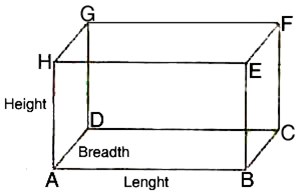If the length of each edge of a cube is 'a' units, then:

Lateral surface area = 4 x (edge)2

Total surface area = 6 x (edge)2

Volume = (edge)3

Diagonal of a cube =  x edge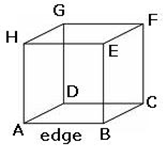Watch a problem solving video below.

Chapter 13 - Surface Areas and Volumes - Ex 13.1

Practise the formula to be used for finding the surface area of a cuboid. Study with the CBSE Class 10 Maths solutions by your side to find out how to calculate the inner surface area of a cylindrical object. Also, learn to compute the total surface area of an object using chapter concepts.

Chapter 13 - Surface Areas and Volumes - Ex 13.2

Through the NCERT solutions for Chapter 13, you’ll be able to find the volume of solids of various shapes. These objects can be a combination of solid shapes such as a hemisphere and a cone or a triangle and a cylinder.

Chapter 13 - Surface Areas and Volumes - Ex 13.3

In the NCERT solutions of the Chapter 13 exercise, you’ll learn to find the radius of a sphere and the height of a cylinder. Based on the available data, you will be able to calculate the height of a shape in real situations such as finding the height of a well.

Chapter 13 - Surface Areas and Volumes - Ex 13.4

Revise the CBSE Class 10 Maths exercises and solutions from this chapter to calculate the capacity of objects. You can do so by calculating the volume of the shape of the solid object. Through the solutions, you’ll learn to use this information to calculate the cost of covering an object with a metal sheet or wrapper.

Chapter 13 - Surface Areas and Volumes - Ex 13.5

How many rounds of wire will be needed to cover a cylinder of a given dimension? Find this answer and more in the NCERT solutions by Maths experts. Also, revise calculations involving the volume of a double cone, a cistern, and more.

NCERT Surface Areas and Volumes Class 10 Videos

NCERT Surface Areas and Volumes Class 10 Revision Notes

NCERT Surface Areas and Volumes Class 10 Most Important Questions

NCERT Surface Areas and Volumes Class 10 MCQ

NCERT Surface Areas and Volumes Class 10 Short Answer Questions

List of sub chapters

Class 10 Maths Surface area combination of solids

Class 10 Maths Volume combination of solids

Class 10 Maths Conversion of solids

Class 10 Maths Frustum of a cone

NCERT solutions for Class 10 Maths Chapter 14 Statistics

Study with ease the concept of grouped and ungrouped data in Statistics. Without taking any stress, you can now revise cumulative frequency curves with our expert solutions for this chapter. Learn how to find the mean, mode, or median of grouped data from our NCERT solutions. Also, learn to solve questions on Statistics by understanding the concept of cumulative frequency distribution and get a proper final solution.

Three measures of central tendency are:

i. Meanii. Medianiii. Mode

The arithmetic mean, also called the average, is the quantity obtained by adding all the observations and then dividing by the total number of observations.

Arithmetic mean may be computed by anyone of the following methods:

Direct method

Short-cut method/Assumed mean method

Step-deviation method

Direct method of finding mean:

If a variant X takes values x1, x2, x3....  xn with corresponding frequencies f1, f2, f3 ,... fn respectively, then arithmetic mean of these values is given by:Class mark = ½ (Upper class limit + Lower class limit)

Short-cut method/ assumed mean method of finding mean:

Let x1, x2....,xn be values of a variable X with corresponding frequencies f1, f2, f3....,fn respectively. Let A be the assumed mean, di = (xi - A). Then:Note that in case of continuous frequency distribution, the values of x1, x2,  x3 ..., xn  are taken as the mid-points or class-marks of the various classes.

Watch a video below.

Chapter 14 - Statistics - Ex 14.1

Revise Statistics lessons with TopperLearning’s Class 10 Maths solutions. Can you find the mean number of plants in a house or the mean daily wages of workers in a factory? You will be able to, with the exercise solutions provided by our experts.

Chapter 14 - Statistics - Ex 14.2

In the CBSE Class 10 Maths book NCERT solutions for Chapter 14, learn how to calculate the class mark as per the given data. Learn to apply the mode formula to find the modal lifetime of objects like electrical components. Revise terms such as class size, lower limit, etc.

Chapter 14 - Statistics - Ex 14.3

Use TopperLearning’s NCERT solutions to understand data analysis using statistical methods such as step deviation method. Revisit lessons on calculating the mean, mode and median of the given. Understand cumulative frequencies and class intervals while studying about data analysis.

Chapter 14 - Statistics - Ex 14.4

In the chapter exercises, you will find questions on representing data in the form of graphs. Learn to draw ogives using data from a cumulative frequency distribution table. Refer our solved CBSE Class 10 Maths NCERT textbook questions  to review the correct answers during Maths practice.

NCERT Statistics Class 10 Videos

NCERT Statistics Class 10 Revision Notes

NCERT Statistics Class 10 Most Important Questions

NCERT Statistics Class 10 MCQ

NCERT Statistics Class 10 FIB

NCERT Statistics Class 10 Short Answer Questions

List of sub chapters

Class 10 Maths- Arithmetic mean of grouped data

Class 10 Maths- Mode of grouped data

Class 10 Maths- Median of grouped data

Class 10 Maths- Relation between central tendencies

Class 10 Maths- Cumulative frequency curve

NCERT solutions for Class 10 Maths Chapter 15 Probability

NCERT Class 10 Maths Chapter 15 includes detailed answers on probability-based questions. These class 10 maths solutions will teach you how to solve simple problems based on the concept of theoretical probability.

1. The theoretical probability (also called classical probability) of an event E, written as P (E), is defined asFor two events A and B of an experiment:

If P(A) > P(B) then event A is more likely to occur than event B.

If P(A) = P(B) then events A and B are equally likely to occur.

An event is said to be sure event if it always occur whenever the experiment is performed. The probability of sure event is always one. In case of sure event elements are same as the sample space.

An event is said to be impossible event if it never occur whenever the experiment is performed. The probability of an impossible event is always zero. Also, the number of favourable outcome is zero for an impossible event.

Probability of an event lies between 0 and 1, both inclusive, i.e.,0 ≤ P (A) ≤1.

If E is an event in a random experiment then the event ‘not E’ (denoted by) is called the complementary event corresponding to E.

The sum of the probabilities of all elementary events of an experiment is 1.

For an event E, where the eventrepresenting ‘not E” is the complement of event E.

Watch a problem solving video on Probability, here.

NCERT Probability Class 10 Videos

NCERT Probability Class 10 Revision Notes

NCERT Probability Class 10 Most Important Questions

NCERT Probability Class 10 MCQ

NCERT Probability Class 10 Short Answer Questions

List of sub chapters

Class 10 Maths- Classical approach to probability

Class 10 Maths- Applications of probability

CBSE Class 10 Chapter-wise Marks Weightage

Plan your Maths revision by understanding the weightage of marks for each chapter. Allot more time to grasping concepts that have high weightage. Study each CBSE Class 10 Maths chapter with TopperLearning’s NCERT solutions and other study resources to gain the skills to score top marks.

Course Structure CBSE Class 10

 Units Unit Name Marks I NUMBER SYSTEMS 06 II ALGEBRA 20 III COORDINATE GEOMETRY 06 IV GEOMETRY 15 V TRIGONOMETRY 12 VI MENSURATION 10 VII STATISTICS & PROBABILTY 11 Total 80

CBSE Class 10 Maths – Standard and Basic Question Paper Design (2021-2022)

The CBSE Board introduces changes in the question paper pattern from time to time. Take a look at the latest question paper pattern for CBSE Class 10 Maths. You will feel more confident when you appear for your exam if you are aware of the paper pattern.

MATHS-STANDARD QUESTION PAPER DESIGN CBSE CLASS 10 (2021-22)

Time: 3 Hours

Max. Marks: 80

 Sr. No. Typology of Questions Total Marks % Weightage (approx.) 1 Remembering: Exhibit memory of previously learned material by recalling facts, terms, basic concepts, and answers. Understanding: Demonstrate understanding of facts and ideas by organizing, comparing, translating, interpreting, giving descriptions, and stating main ideas 43 54 2 Applying: Solve problems to new situations by applying acquired knowledge, facts, techniques and rules in a different way. 19 24 3 Analysing : Examine and break information into parts by identifying motives or causes. Make inferences and find evidence to support generalizations Evaluating: Present and defend opinions by making judgments about information, validity of ideas, or quality of work based on a set of criteria. Creating: Compile information together in a different way by combining elements in a new pattern or proposing alternative solutions 18 22 Total 80 100

 Internal Assessment Marks 20 Pen Paper Test and Multiple Assessment (5+5) 10 Marks Portfolio 05 Marks Lab Practical (Lab activities to be done from the prescribed books) 05 Marks

MATHS-BASIC QUESTION PAPER DESIGN CBSE CLASS 10 (2021-22)

Time: 3 Hours

Max. Marks: 80

 Sr. No. Typology of Questions Total Marks % Weightage (approx.) 1 Remembering: Exhibit memory of previously learned material by recalling facts, terms, basic concepts, and answers. Understanding: Demonstrate understanding of facts and ideas by organizing, comparing, translating, interpreting, giving descriptions, and stating main ideas 60 75 2 Applying: Solve problems to new situations by applying acquired knowledge, facts, techniques and rules in a different way. 12 15 3 Analysing : Examine and break information into parts by identifying motives or causes. Make inferences and find evidence to support generalizations Evaluating: Present and defend opinions by making judgments about information, validity of ideas, or quality of work based on a set of criteria. Creating: Compile information together in a different way by combining elements in a new pattern or proposing alternative solutions 8 10 Total 80 100

 Internal Assessment Marks 20 Pen Paper Test and Multiple Assessment (5+5) 10 Marks Portfolio 05 Marks Lab Practical (Lab activities to be done from the prescribed books) 05 Marks

## Benefits of NCERT Solutions Class 10 Maths

Our experts use their decades of experience to prepare NCERT solutions. These have been giving students the right support and guidance when dealing with exam fear. TopperLearning allows you to improve your speed and accuracy while solving Maths problems by providing you with the best study materials such as NCERT Solutions from some of the best teachers in the country. Our teachers put in tremendous efforts to ensure that you receive the NCERT solutions for Class 10 Maths as per the most recent CBSE guidelines.

Through our NCERT solutions for Class 10 Maths, our experts increase your logical thinking capabilities. In this way, when you read a question in your exam, instead of directly assuming answers or solutions to the questions, you can use your acquired skills to come up with the best method to solve problems. Once you identify the method, half your work is done as you have a perfect solution ready for any Maths Class 10 exam question.

With clarity on the method for solving a problem using NCERT Solutions, you are equipped to write the correct steps and a  Mathscomplete answer. If your approach to solving a Maths problem is correct, you will get marks for the steps even if you happen to make mistakes with the calculations.

## Why should I get TopperLearning NCERT Solutions for Class 10?

At TopperLearning, you will get chapter-wise NCERT solutions for Class 10 Maths along with concept notes. Instead of merely providing solutions, our experts also add concept notes to help you understand the solutions better. You can follow a step-by-step learning approach by studying one chapter at a time. If you need help to explore and understand the concepts with proper solutions, you can check out our Class 10 Maths video lectures and Class 10 Maths revision notes. Evaluate your knowledge of the topics in each chapter with our online tests. Also, check out the systematic weekly practice tests that can be accessed through our subscription plans

Times have changed, and the best in e-learning solutions is now available to you anytime, anywhere. You no longer have to wait to go to a teacher in a physical classroom to get your doubts cleared. TopperLearning’s experts are available online through our ‘Undoubt’ section with proper solutions to quickly clear your doubts related to Maths Class 10. During your vacation, you can continue your self-study by going through all the Class 10 important notes which are available for free on the TopperLearning online study portal.

## Frequently Asked Questions (FAQs) - CBSE Class 10 Mathematics

1.    Why should one go through the NCERT Solutions for Class 10 Maths?

NCERT books are recommended by CBSE, so it is very important that you get enough practice. We also have sample papers, RS Aggarwal and RD Sharma solutions to help you get maximum practice.

2.    How can I assess my progress?

TopperLearning has enough revision material that will help you gauge your progress and performance in each test.

3.    Why should I use TopperLearning to prepare for the CBSE Class 10 exam?

TopperLearning has 950+ video lessons, 200+ revision notes, 8500+ questions and 15+ sample papers (including CBSE Class 10 sample papers 2019) for CBSE students. The study material at TopperLearning is designed to give you clarity on the concepts that you study in school.

4.    Can I clear my doubts for NCERT solutions?

You can ask questions across topics and our experts will get in touch with you within 24 hours.

5.    Are the NCERT solutions elaborate?

Yes, the NCERT solutions not only have answers but also concept notes for a better understanding of students.

6.       Is CBSE Class 10 Maths difficult?

You may find certain chapters in Class 10 difficult if your concepts are not clear. Gain conceptual clarity with thorough Maths practice and you will be more comfortable with solving Maths problems.

7.       How to get above 95% in Class 10 (CBSE) with 1-2 months of preparation?

Revise the entire CBSE Class 10 Maths syllabus at least once. Focus on thorough preparation of chapters that have high weightage. Put in your best efforts and you can score reasonably good marks. Based on your grasping power and preparation time, you can chart a plan to work towards scoring 95% in the exam.

8.       How to get 100/100 marks in Maths CBSE Class 10?

Conceptual clarity and thorough revision of the CBSE Class 10 Maths syllabus concepts can help you get 100/100 marks.

9.       How to pass the Maths exam in the CBSE Class 10?

If you are anxious about your exam, don’t worry. Revise Maths lessons using our chapter notes, videos, and textbook solutions. Gain the confidence to beat anxiety and confidently appear for your exam by taking self-assessments and mock exams with Maths sample paper for CBSE Class 10 and solutions.

10.   How to top the Maths 10th Class CBSE board exam?

Take advantage of online learning resources like chapter notes, concept videos, NCERT solutions, and more. Assess your skills and update your revision study plan to include topics that you struggle with. With hard work and perseverance, you can prepare to top the CBSE Class 10 Maths board exam.

11. Which chapters in NCERT Solutions For Class 10 Maths are important to prepare for the exams?

NCERT Solutions for Class 10 Maths has fifteen chapters, namely:

• Chapter 1 - Real Numbers
• Chapter 2 - Polynomials
• Chapter 3 - Pair Of Linear Equations In Two Variables
• Chapter 4 - Quadratic Equations
• Chapter 5 - Arithmetic Progression
• Chapter 6 - Triangles
• Chapter 7 - Coordinate Geometry
• Chapter 8 - Introduction To Trigonometry
• Chapter 9 - Some Applications Of Trigonometry
• Chapter 10 - Circles
• Chapter 11 - Constructions
• Chapter 12 - Areas Related To Circles
• Chapter 13 - Surface Areas And Volumes
• Chapter 14 - Statistics
• Chapter 15 - Probability

Out of these 15, Chapters 3, 5, 6, 7, 8, 9, 13, and 14 are the most important ones in the Class 10 Maths Solutions book. The CBSE question paper is generally divided into four sections: A, B, C, and D and 40 questions are asked from the syllabus. However, the majority of the questions come from these chapters.

The marks allotment for each chapter is mentioned below:

Chapter 3: Pair of Linear Equations in Two Variables = 8 Marks

Chapter 5: Arithmetic Progression = 5 Marks

Chapter 6: Triangles = 9 Marks

Chapter 7: Coordinate Geometry = 6 Marks

Chapter 8: Introduction to Trigonometry = 6 Marks

Chapter 9: Some Applications of Trigonometry = 6 Marks

Chapter 13: Surface Areas and Volumes = 7 Marks

Chapter 14: Statistics = 8 Marks

12. Where can I get the chapter wise answers of the NCERT Solutions for Class 10 Maths?

You can get chapter-wise answers to the questions asked in the NCERT solutions for CBSE Class 10 Mathematics questions in the pdf. You can download the pdf wise chapters from the official website of NCERT or from our page to solve your problems.

The answers to the questions in the Class 10 Maths Solutions are the best learning sources available to students. These CBSE NCERT Solutions for Class 10 Maths 2021-22 assist students in developing a more in-depth grasp of subjects presented in the textbook. Students can assess their degree of preparation and subject comprehension by solving textbook questions. In addition, the answers to these questions in the books can assist students in quickly resolving their difficulties.

The NCERT Solutions for Class 10 Maths list contains all chapter-by-chapter answers to the questions in the NCERT Book for Class 10 Maths, written clearly and logically while adhering to the textbook's objectives. Candidates can use the NCERT Solutions for Class 10 as additional references and study tools. In addition, students would undoubtedly benefit from practising NCERT textbook exercise solutions as part of their test preparation.

13. How can I download chapter wise NCERT Solutions for Class 10 Maths?

You can download chapter wise NCERT solutions for CBSE Class 10 Mathematics from their official website. You can follow the steps below to download all the chapters you want.

• Go to the official website of NCERT ( https://ncert.nic.in/ )
• Type "Textbooks PDF (I-XII)" in the search box and press on "Enter".
• A page will appear.
• On this page, select "Class", "Subject", and "Book Title" as asked and then click on "Go".
• Another page will appear. On the left side, you will notice the chapter numbering of the subject you choose, and on the right side, you will find the front page of any pdf you select.
• Now click on the chapter about whose Class 10 Maths Solutions you need. Finally, click on "Open" beside the chapter number to view the pdf on the right side.
• Now on the top of the pdf, you will see a download option beside the "Print" option, click on that, and your pdf will be downloaded.

You can also get access to the pdf's solution on TopperLearning. For that, you need to visit our website https://www.topperlearning.com/ and choose the class, subject, and chapter number to get the NCERT Solutions for Class 10 Maths or any other topic you want to look for.